# CodingBobby | Phase Space

The following graphics show the phase space of specific differential systems which contain periodically appearing attractors. Unlike the typical three-dimensional strange attractors, they don’t have a single bounding box no point will ever escape but many more, if not infinitly many covering the entire two-dimensional plane.

These systems can be generally written as

$\dot{x} = -\,f(y)$ $\dot{y} = \,f(x)$

where $$f$$ is an arbitrary function dictating the system’s behaviour. Planes are centered at $$(0,0)$$ unless otherwise stated.

Inspiration for this project was Cliff Pickover’s book CPCB1 – again, I know; it is like Gabriel’s Horn of chaos for me.

## Plane Tesselation

Perhaps the simplest forms $$f$$ can take in order to produce a repeating pattern is this:

$f(u) = \sin(u)$

And here is the result: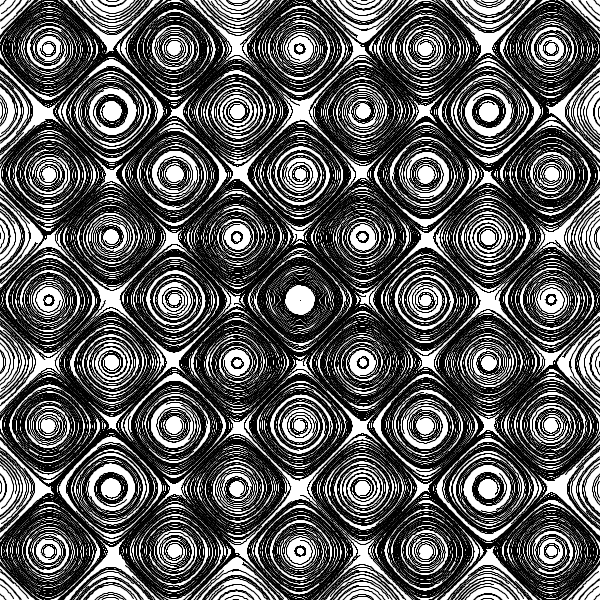It might already look chaotic but that is mostly because the the sampled points do not align with the pattern. Let’s add another term:

$f(u) = \sin(u) + \sin^2(u)$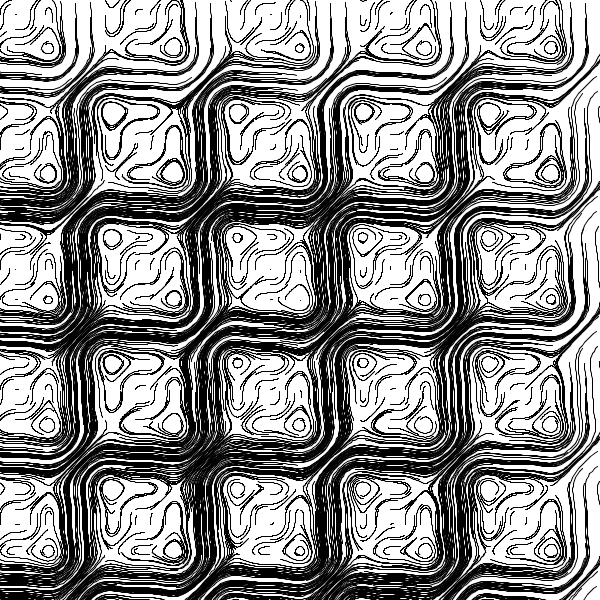Because these patterns actually get a bit repetitive, I won’t show the following images with the same scale but zoom on more interesting regions. Here, the frequency $$u$$ is modulated with another periodic function:

$f(u) = \sin\left(u + \sin(u)\right)$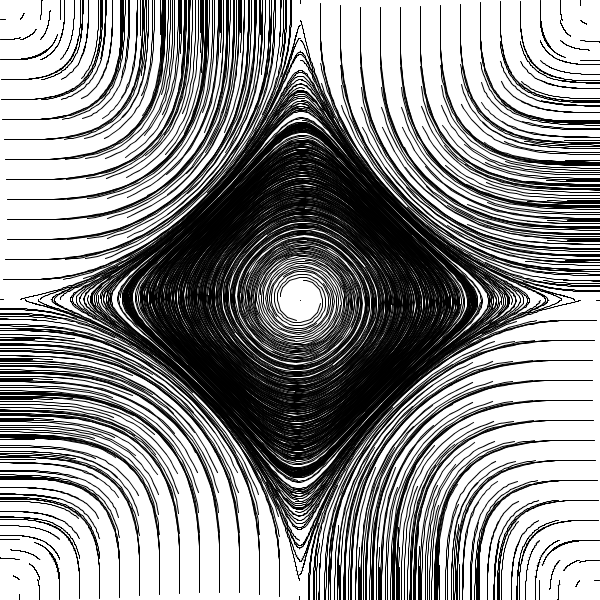We can change the impact of a specific term or modify its frequency by introducing a factor $$\varrho$$:

$f(u) = \sin\left(u + \sin(\varrho\,u)\right)$ $\varrho = 5$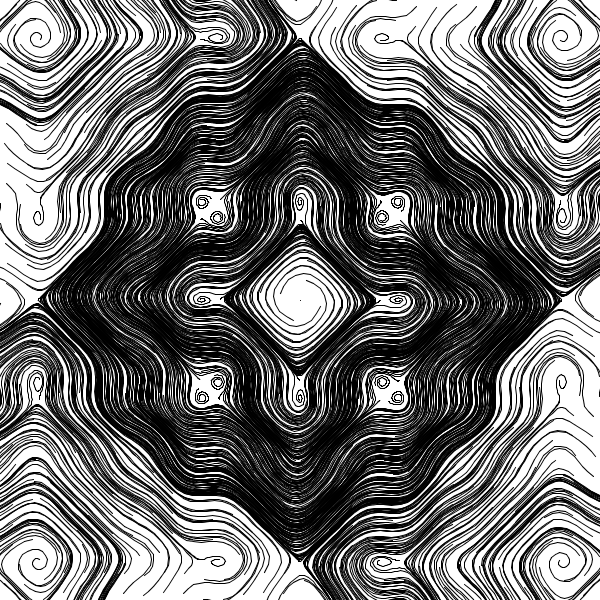Longer terms allow asymmetric use of $$\varrho$$:

$f(u) = \sin\left(u + \sin^2(\varrho\,u)\right) + \sin\left(\varrho\,u + \sin^2(u)\right)$ $\varrho = 0.3$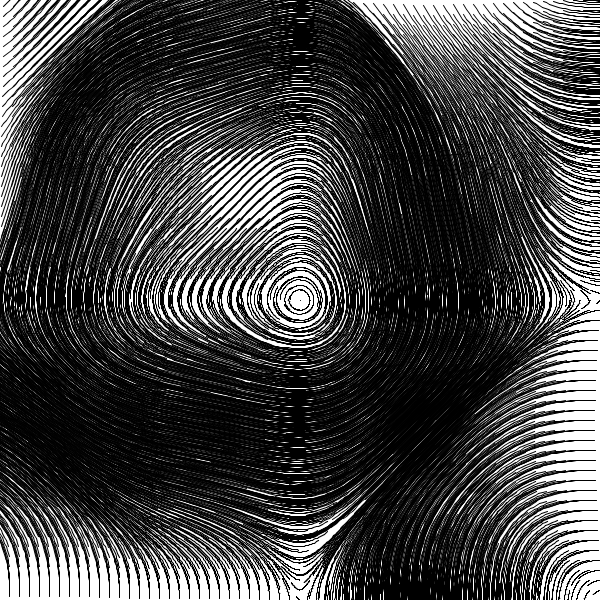Even more chaotic behaviour happens with powers:

$f(u) = \sin\left(\varrho^u + \sin^2(\varrho\,u)\right)$ $\varrho = 3.6$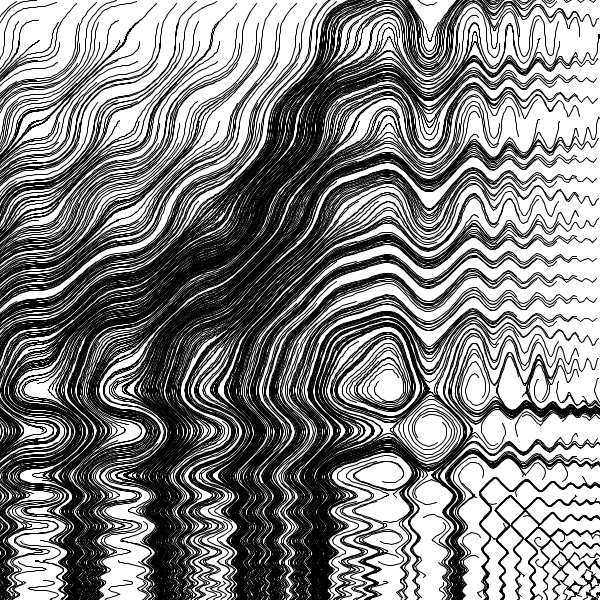Other modulation functions are also interesting. $$\tan$$ creates unexpectedly chaotic shapes:

$f(u) = \sin\left(u + \tan^2(\varrho\,u)\right)$ $\varrho = 1.5$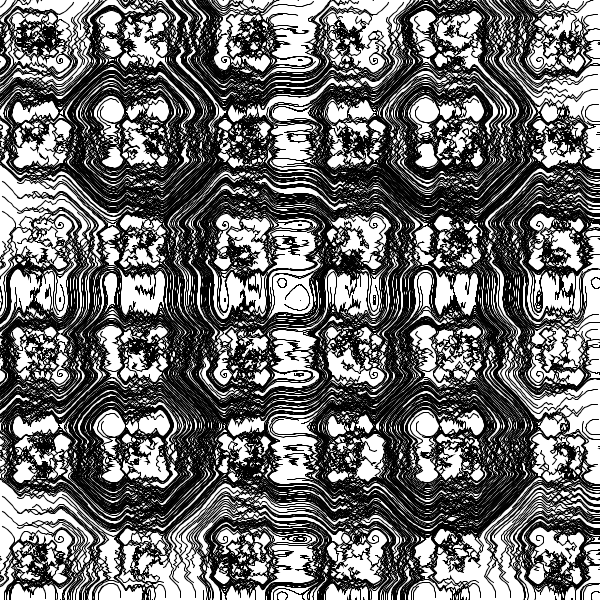A magnification of the central swirl: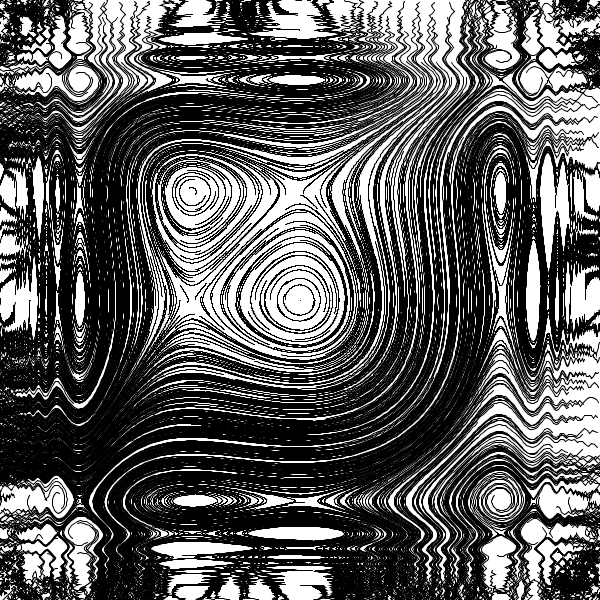## Randomness

Some tesselations get finer and finer towards larger $$x$$ and $$y$$ until their details hit a limit where the step size $$\dot{x}$$ and $$\dot{y}$$ respectively is greater than the period of the pattern. Because the points are moving too fast, they overshoot their correct trajectory and get trapped in a neighbouring attractor, which they leave again shortly afterwards. As a result, they walk seemingly randomly across the plane.

$f(u) = \sin\left(u^3 + \sin^2(\varrho\,u)\right)$ $\varrho = 3$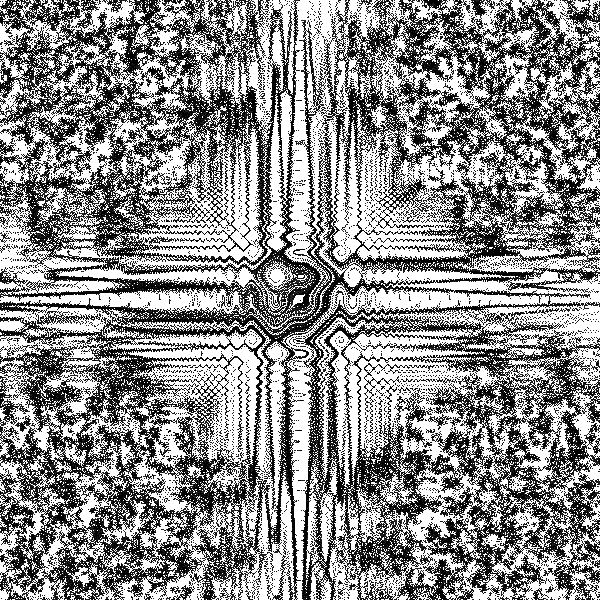When the velocity is reduced, the non-random area extends, resolving finer details of the true phase space:Here is a magnification of the transition between non-random to random walk (in the bottom right quadrant):The “moment” when a trajectory is deflected towards another attractor is clearly visible. These are the same points but they are moving with half the velocity (like in the larger images above):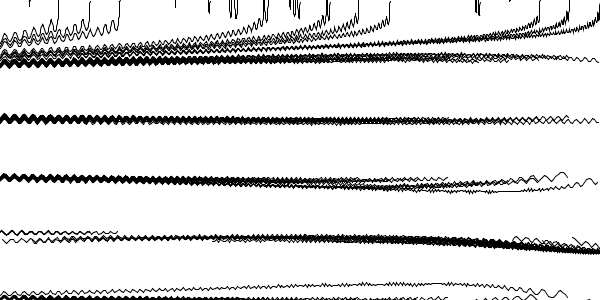1. C.A. Pickover, 1990. "Computers, Pattern, Chaos, and Beauty". St. Martin’s Press. ISBN: 0312041233.

<- 🕸 💍 ->